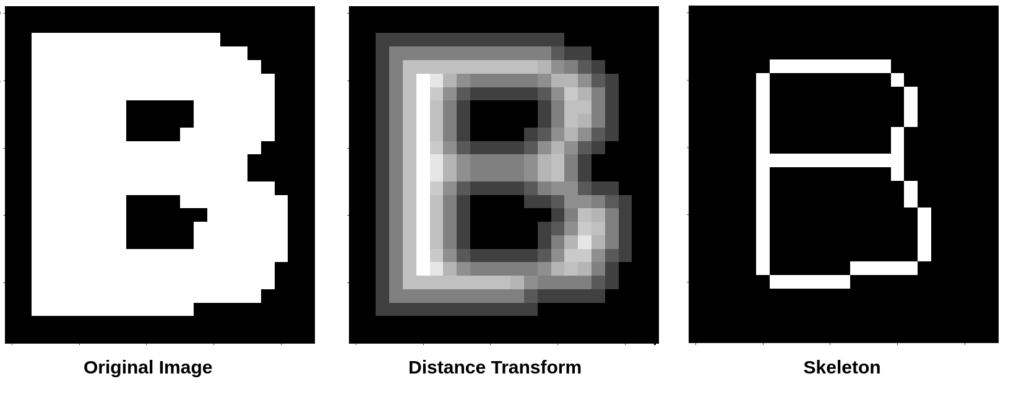## Stroke width identification algorithm for text like regions

In character recognition, the distance between two edges of a stroke, measured perpendicular to the stroke centerline is called the stroke width. Take a look at the below image. The length of lines marked in Red gives the stroke width of the character. There are quite a few ways to identify the stroke width, In …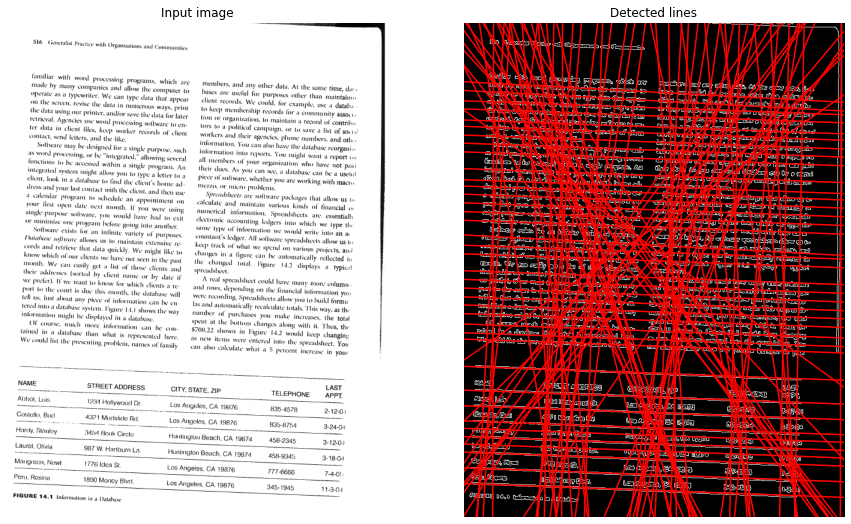## Skew Detection and Correction of Document images using Hough Transform

In this post I will be talking about how we can use Hough Transform to detect and correct Skewness of a document image. There have been many research papers published around this problem and it keeps getting published even today on various journals mainly because its still largely an unsolved problem. I had previously written …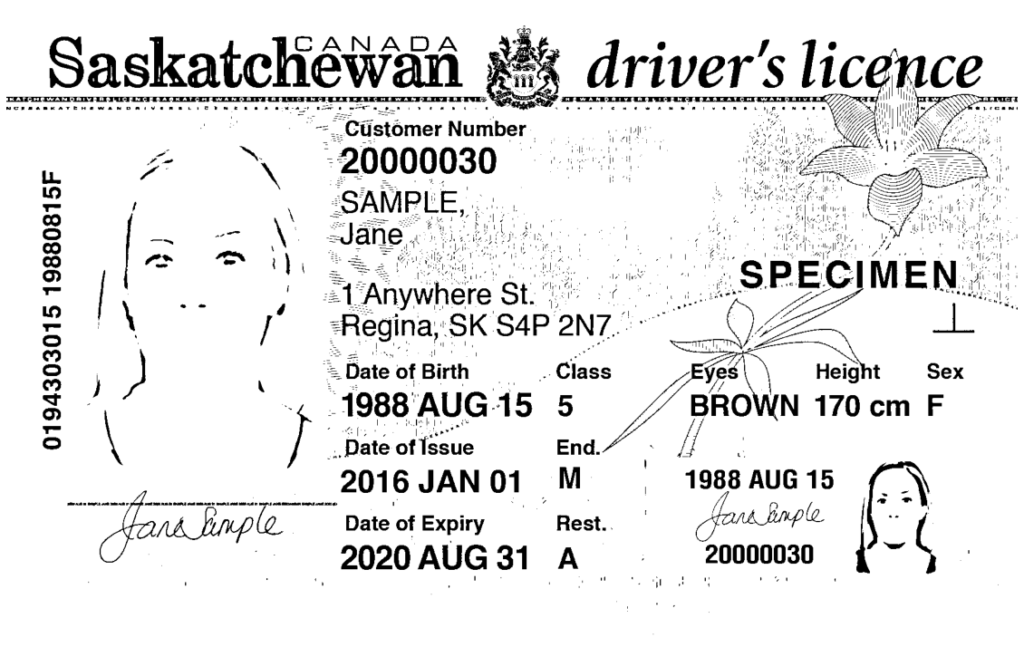## Pre processing for Detecting text in images

One of the important steps in OCR is the thresholding process. It helps us in separating the text regions (foreground) from the background. If you apply a thresholding algorithm like OTSU or Sauvola, you might end up with a lot of noise. Some of your text regions may even get categorized as background. Take a …

## All Tesseract OCR options

This is for my reference and this might come in handy for others too. All Tesseract options \$ tesseract –help-extra CLI Examples Command Example Notes tesseract sample_images/image2.jpg stdout To print the output to standard output tesseract sample_images/image2.jpg sample_images/output By default the output will be named outbase.txt. tesseract sample_images/image2.jpg sample_images/output -l eng -l is for language. …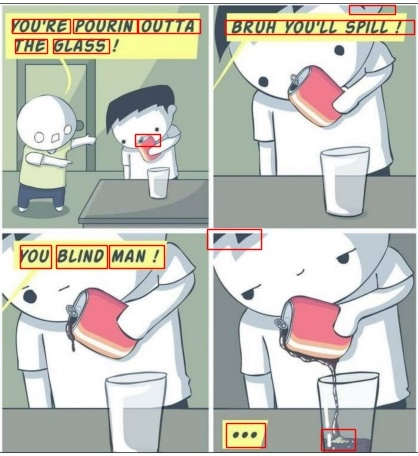## Extracting Text Regions from an image using Geometric properties

The problem of building systems that mimic human behavior is not an easy one to solve. Neural Nets solve those problems in one way but if someone thinks that it’s the right way doesn’t know enough about Artificial Intelligence. When you show a child a picture of a dog, just one picture, the child can …## Otsu’s method for image thresholding explained and implemented

The process of separating the foreground pixels from the background is called thresholding. There are many ways of achieving optimal thresholding and one of the ways is called the Otsu’s method, proposed by Nobuyuki Otsu. Otsu’s method is a variance-based technique to find the threshold value where the weighted variance between the foreground and background …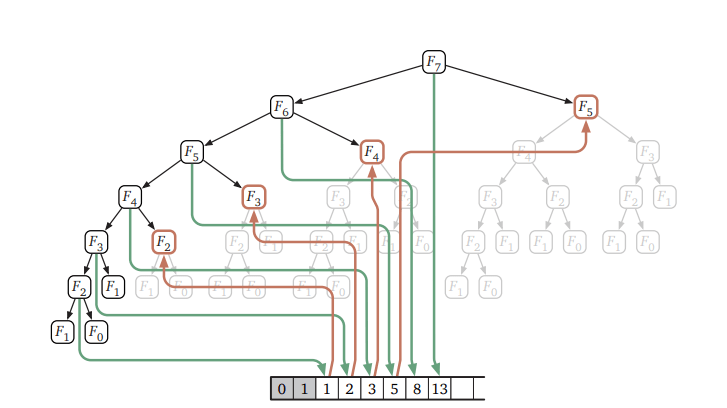## Fast nth Fibonacci number algorithm

Definition: The Fibonacci sequence is defined by the equation, where $$F(0) = 0$$, $$F(1) = 1$$ and $$F(n) = F(n-1) + F(n-2) \text{for } n \geq 2$$. This gives us the sequence 0,1,1,2,3,5,8,13 … called the Fibonacci Sequence. This post is about how fast we can find the nth number in the …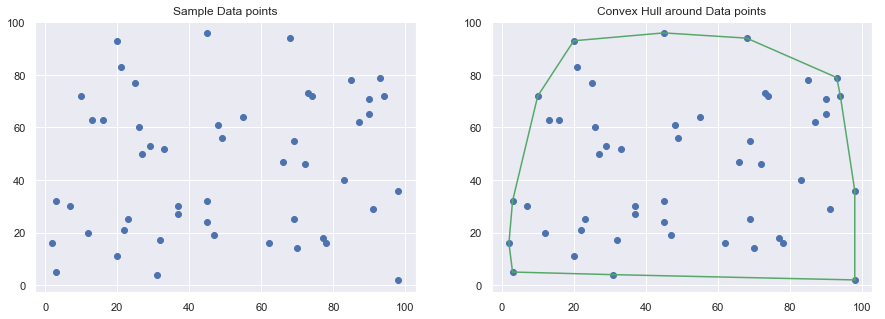## Understanding Graham scan algorithm for finding the Convex hull of a set of Points

Convex Hull is one of the fundamental algorithms in Computational geometry used in many computer vision applications like Collision avoidance in Self Driving Cars, Shape analysis and Hand Gesture-recognition, etc. By Definition, A Convex Hull is the smallest convex set that encloses a given set of points. For Example, Given a set of points P …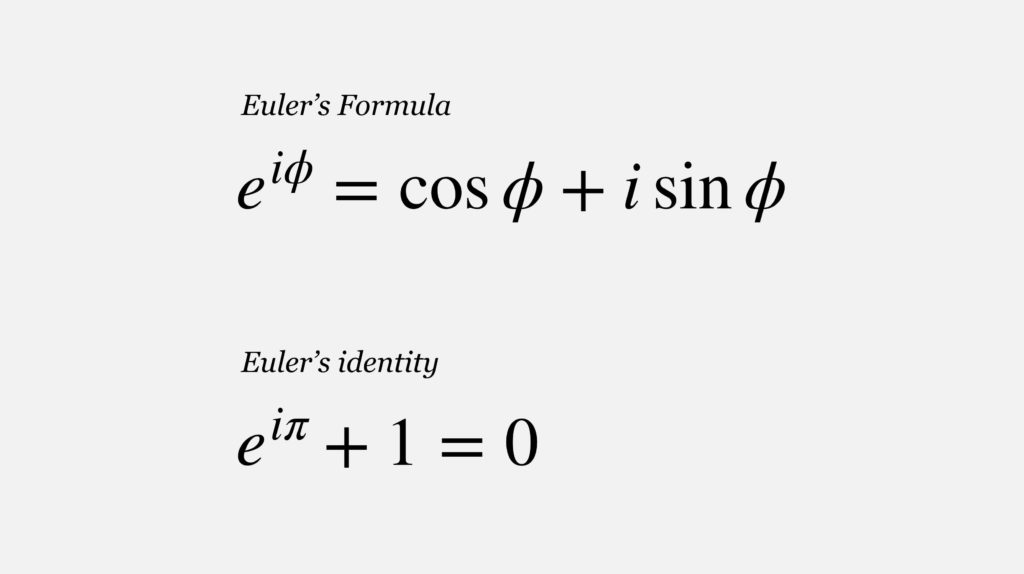## Deriving the famous Euler’s formula through Taylor Series

Euler’s formula is often coined the most remarkable formula in mathematics. It combines the seemingly unrelated exponential functions, imaginary numbers, and trigonometric functions into a single aesthetically pleasing and beautiful equation. We see five numbers (e, i, π, 0, and 1) that we are familiar with as well as three simple operations (exponentiation, multiplication, and …